# 逆元讲解（三种方法）

【同余的定义】：【同余的主要性质】：

(a+b)%d=(a%d+b%d)%d
加减乘除都能分开写
要注意的是减法，因为减法可能会减出来负值所以可以这样写（a-b+mod）%mod；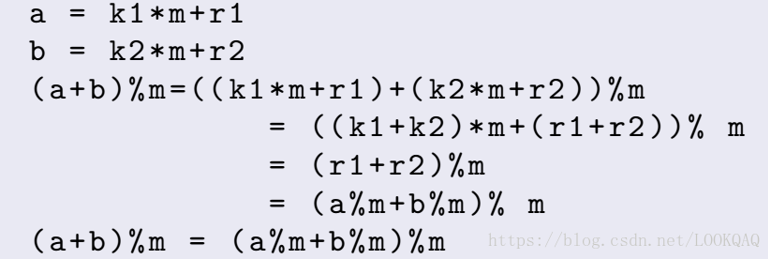【逆元】
（1）定义：
就是一个数的倒数，那为什么要求一个数的倒数：比如a/b这个时候b的值特别大，就是导致double精度不够所以我们要将a/b换成a*c，其中c^-1=b.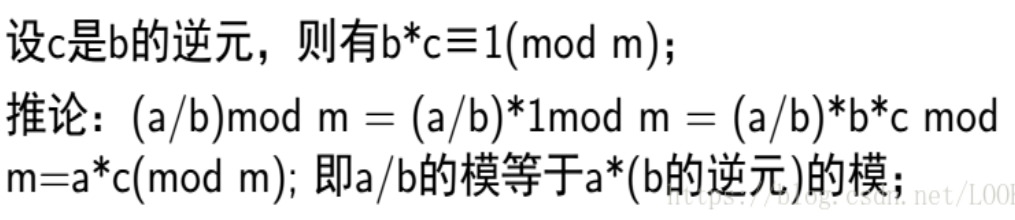【费马小引理求解逆元】：（易知费马定理是有限制的：a与p要互质）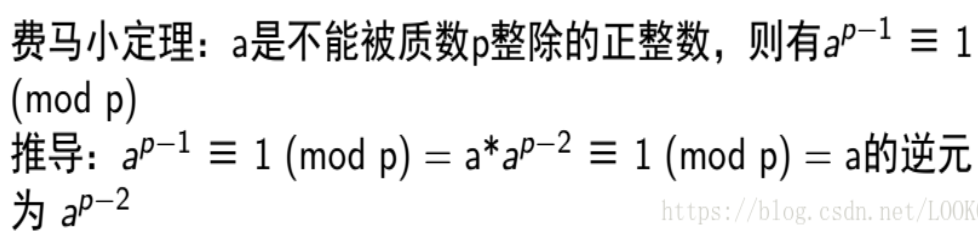1 long long quickpow(long long a,long long b){
2  if(b<0)  return 0;
3  long long ret=1;
4  a%=mod;
5  while(b){
6   if(b & 1 ) ret = ( ret *a ) % mod
7   b>>=1;
8   a = (a * a)% mod;
9  }
10  return ret;
11 }
12 long long inv(long long a){
13  return quickpow(a,mod-2);
14 }

【扩展欧几里得算法求逆元】：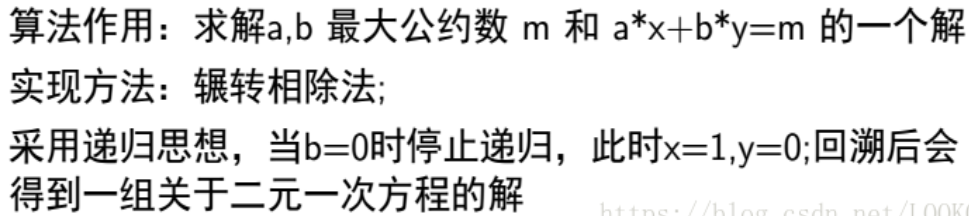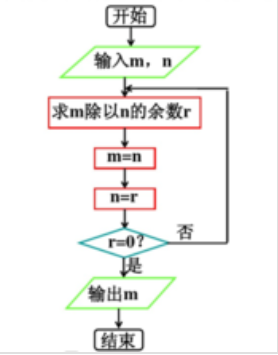（2）扩展欧几里得算法的证明：（这种方法也要求a和m互质）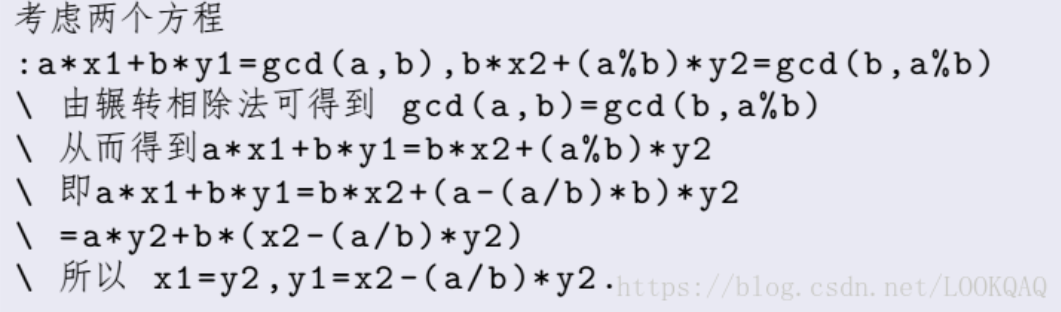（3）求解逆元：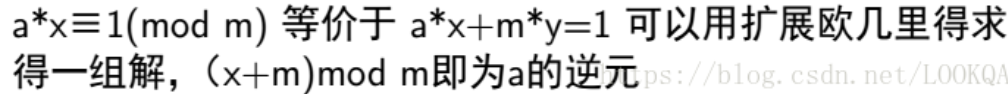（4）代码实现：
 1 #include <iostream>
2 #include <stdio.h>
3 #include <stdlib.h>
4 #include <ctype.h>
5 #include<string.h>
6 #include <math.h>
7 #include<algorithm>
8 using namespace std;
9 typedef long long ll;
10 void extgcd(ll a,ll b,ll& d,ll& x,ll& y)
11 {
12     if(!b)
13     {
14         d=a;
15         x=1;
16         y=0;
17     }
18     else
19     {
20         extgcd(b,a%b,d,y,x);
21         y-=x*(a/b);
22     }
23 }
24 int ModularInverse(int a,int b)
25 {
26
27     ll d,x,y;
28     extgcd(a,b,d,x,y);
29     return d==1?(x+b)%b:-1;  //返回的结果就是(1/a)mod(b)的结果
30     // complete this part
31 }
32 int main()
33 {
34     printf("%d\n",ModularInverse(2,3));//结果是2
35     /*
36     2*x+3*y=1mod(3)  //那个x就是(1/2)mod(3)的结果，y的话不用管，因为3*y取模于3都是0
37     */
38     return 0;
39 }

（3）、

i-1≡-k*r-1(mod p)

i-1≡-(p/i)*(p%i)-1(mod p)

 1 //1、线性求逆元
2 int inv[MAXN];
3 void INV(int a,int p)//线性求到a的逆元
4 {
5     inv = 1;
6     for (int i=2; i<=a; ++i)
7         inv[i] = (-(p/i))*inv[p%i]%p;
8 }
9
10 //2、单独求某个值的逆元
11 int INV(int a)//线性求a的逆元
12 {
13      if (a==1) return 1;
14      return ((-(p/a)*INV(p%a))%p);
15 }

posted @ 2019-03-23 00:45  kongbursi  阅读(14439)  评论(0编辑  收藏  举报